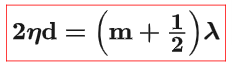# What visible wavelengths of light are strongly reflected from a 390-nm-thick soap bubble?# What visible wavelengths of light are strongly reflected from a 390-nm-thick soap bubble?

You are at the right destination if you are looking for the answer to What visible wavelengths of light are strongly reflected from a 390-nm-thick soap bubble?. In this blog post, you will get 100% accurate and correct answers from experts.

Problem Details:

A soap bubble is essentially a thin film of water surrounded by air. The colors you see in soap bubbles are produced by interference.

• (a) What visible wavelengths of light are strongly reflected from a 390 nm -thick soap bubble?
• (b) What color would a 390 nm thick soap bubble appear to be (green, purple, or yellow)?

Question:

A soap bubble is essentially a thin film of water surrounded by air. The colors you see in soap bubbles are produced by interference. What visible wavelengths of light are strongly reflected from a 390-nm-thick soap bubble? What color would such a soap bubble appear to be?

Solution 1:Result:Solution 2:

Constructive interference in thin films (when the film material has a higher index of refraction):(a)

Refractive index of water, η = 1.33

d = 390 nm (10-9m/1nm) = 390 × 10-9 m

Solving for the wavelength, λ:

λ = 2nd/(m + 1/2)

Calculating  λ when m = 1:

λ = 2nd/(m + 1/2) = 2 × 1.33 × 390 × 10-9 /(1 + 1/2) = 692 × 10-9 m(1nm/10-9m) = 692 nm

Calculating  λ when m = 2:

λ = 2nd/(m + 1/2) = 2 × 1.33 × 390 × 10-9 /(2 + 1/2) = 415 × 10-9 m(1nm/10-9m) = 415 nm

The wavelengths of viscible light that are strongly reflected are 692 nm and 415 nm.

(b)

692 nm wavelength corresponds to color red.

415 nm Corresponds to violet.

Mixing red and violet colors yields purple.

The soap bubble will appear purple.